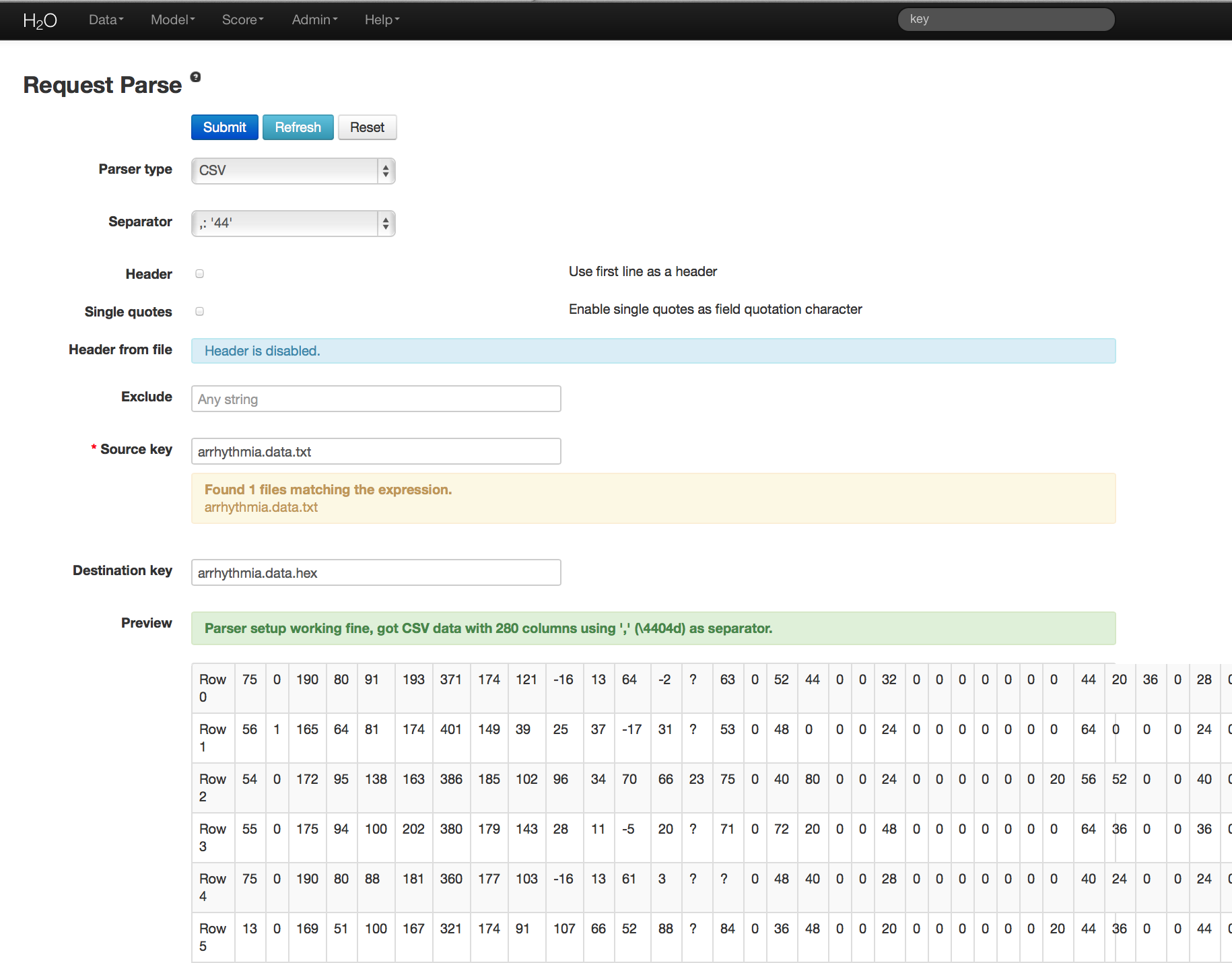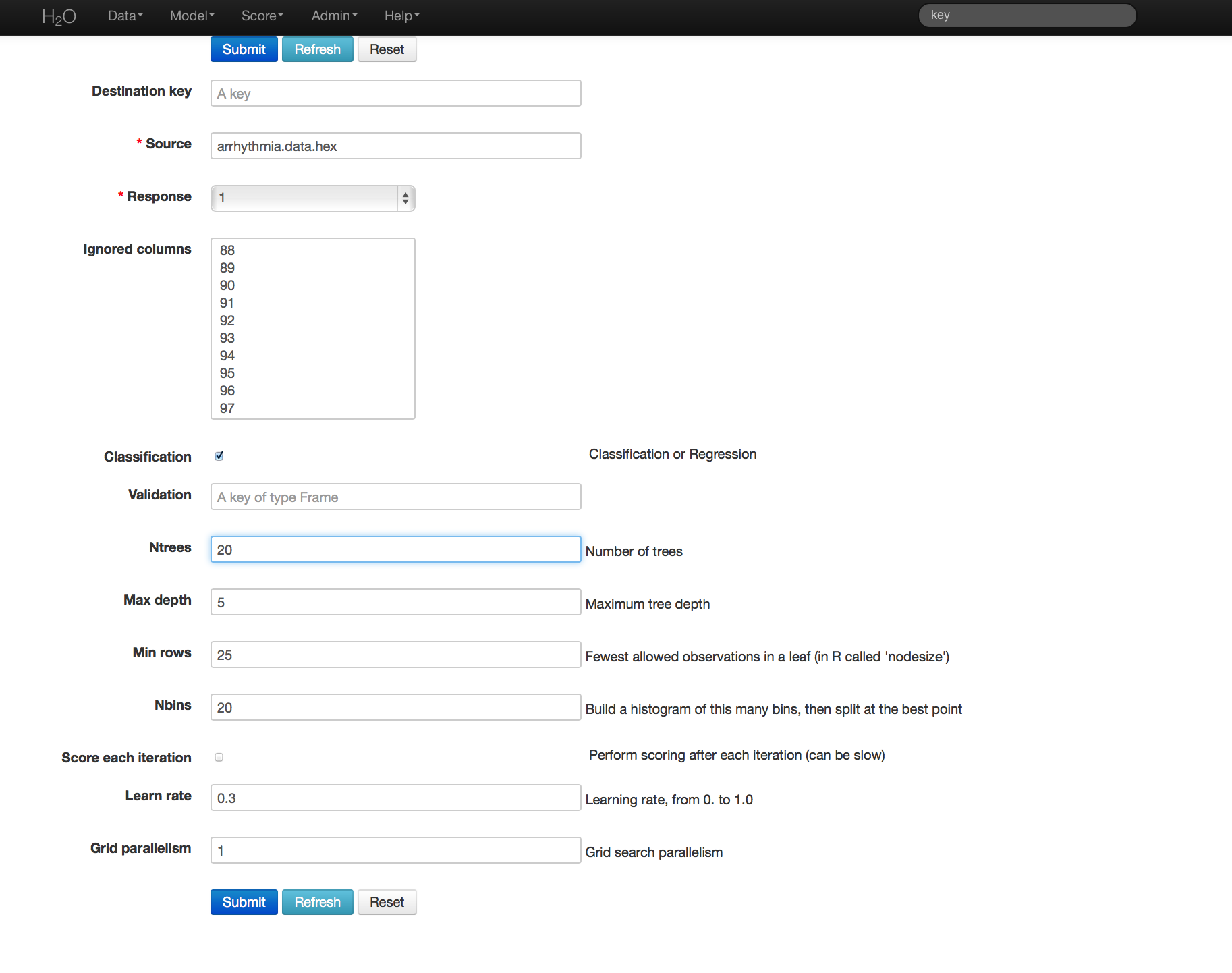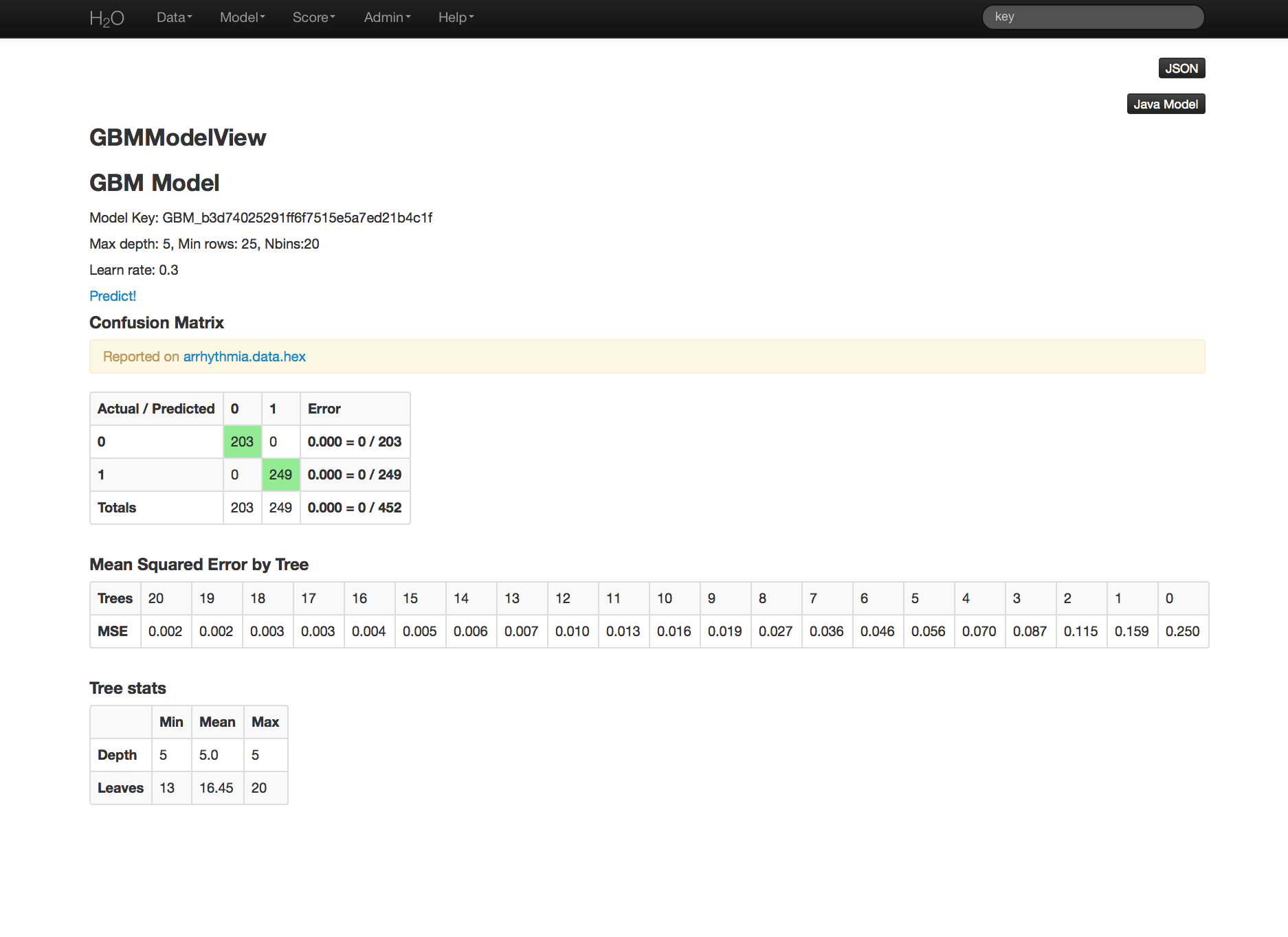# GBM Tutorial¶

The purpose of this tutorial is to walk the new user through a GBM analysis in H2O

Those who have never used H2O before should see the quick start guide for additional instructions on how to run H2O.

## Getting Started¶

This tutorial uses a publicly available data set that can be found at: http://archive.ics.uci.edu/ml/datasets/Arrhythmia

The original data are the Arrhythmia data set made available by UCI Machine Learning repository. They are composed of 452 observations and 279 attributes.

Before modeling, parse data into H2O as follows:

2. User will be redirected to a page with the header “Request Parse”. Select whether the first row of the data set is a header. All other settings can be left in default. Press Submit.
3. Parsing data into H2O generates a .hex key (“data name.hex”)## Building a Model¶

1. Once data are parsed a horizontal menu will appear at the top of the screen reading “Build model using ... ”. Select GBM here, or go to the drop down menu Model and select GBM.
2. In the Source field enter the .hex key for the Arrhythmia data set.
3. In the Response field select the response variable. In this case it is variable 1.
4. In Ignored Columns select the subset of variables that should be omitted from the model. In this case, the only column to be omitted is the index column, 0.
5. Users have the option of Gradient Boosted Classification or Gradient Boosted Regression. GBM is set to classification by default. For this example, the desired output is classification.
6. In Validation enter the hex key associated with a holdout (testing) data set, if results should be applied to a new data set after the model is generated.
7. In Ntrees set the number of trees you would like the model to generate. In this case 20.
8. In Max Depth specify the maximum number of edges between the top node and the furthest node as a stopping criteria. Here the depth of interaction is set to 5.
9. Specify Min Rows to be the minimum number of observations (rows) included in any terminal node as a stopping criteria. In this case 25.
10. Nbins are the number of bins in which data are to be split, and split points are evaluated at the boundaries of each of these bins. As Nbins goes up, the more closely the algorithm approximates evaluating each individual observation as a split point. The trade off for this refinement is an increase in computational time.
11. Learn Rate is a tuning parameter that slows the convergence of the algorithm to a solution, and is intended to prevent overfitting. In this case we set learn rate to .3. (This parameter is often alternatively referred to as shrinkage).## GBM Results¶

GBM output for classification returns a confusion matrix showing the classifications for each group, and the associated error by group and the overall average error. Regression models can be quite complex and difficult to directly interpret. For that reason only a model key is given, for subsequent use in validation and prediction. Both models provide the MSE by tree. For classification models this is based on the classification error within the tree. For regression models MSE is calculated from the squared deviances, as it is in standard regressions.THE END.